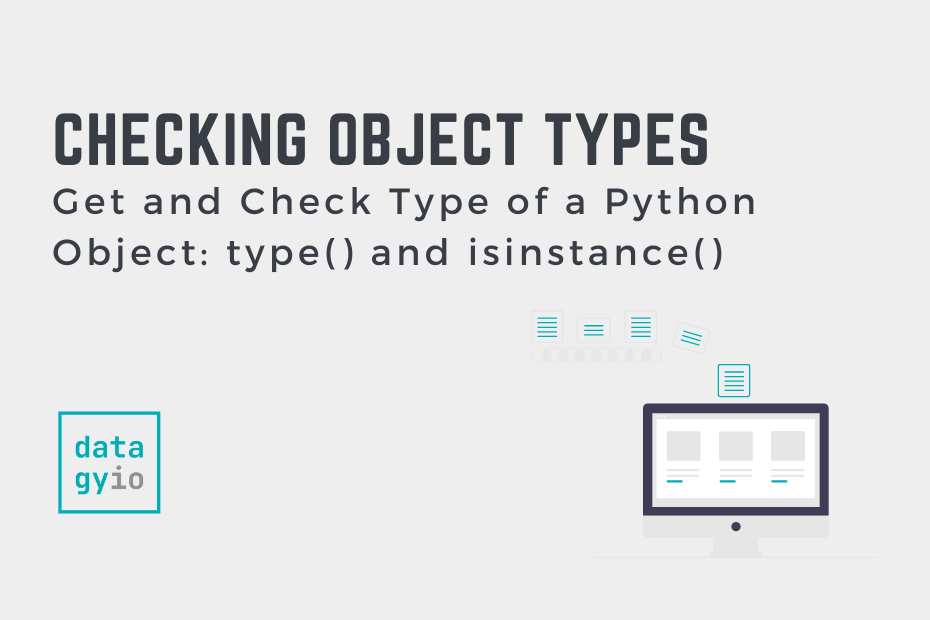# Get and Check Type of a Python Object: type() and isinstance()In this tutorial, you’ll learn how to get and check the type of a Python object using the type() and isinstance() functions. Everything in Python is an object and knowing what the object’s type is allows you to make better-informed decisions about what your code is doing.

By the end of this tutorial, you’ll have learned:

• How to determine the type of an object using the `type()` function
• How to check if an object is an instance of a class using the `isinstance()` function
• How to check if an object is a subclass of another class

## Get the Type of a Python Object with type()

The Python `type()` function is used to return the type of an object that is passed in as an argument. The `type()` function is analogous to the `typeof()` function in other programming languages. You can pass in either the variable referencing an object or the object itself.

Let’s take a look at how to use the `type()` function in Python:

``````# Using the type() Function to Get the Type of an Object
message = 'Welcome to datagy.io!'
number = 342
pi = 3.14

print(f'The type of <message> is: {type(message)}')
print(f'The type of <number> is: {type(number)}')
print(f'The type of <pi> is: {type(pi)}')

# Returns:
# The type of <message> is: <class 'str'>
# The type of <number> is: <class 'int'>
# The type of <pi> is: <class 'float'>``````

The code sample above shows the use the Python `type()` function to provide an overview of the types of different objects.

Similarly, we can use the type function for custom classes. Let’s first create a class, instantiate it and then check the object’s type:

``````# Using the type() Function with Custom Objects
class CustomObject:
pass

obj = CustomObject()
print(type(obj))

# Returns: <class '__main__.CustomObject'>``````

In the code above, we first create a new class: `CustomObject`. We then create an object by instantiating the class and check its type using the `type()` function.

## How to Use Python isinstance() to Check the Type of an Object

The Python `isinstance()` function checks whether or not an object belongs to an object type or a number of types. The function works differently from the `type()` function by checking against a type of variety of types and returns a boolean value.

Let’s take a look at how to use the `isinstance()` function works:

``````# Using the isinstance() Function
message = 'Welcome to datagy.io!'
print(isinstance(message, str))

# Returns: True``````

We can see that the function checks whether the object `message` is of type `str`. Because it is, the function returns `True`.

We can even check against a variety of different types by passing in a tuple of types. Let’s take a look at an example to help clarify this:

``````# Using the isinstance() Function with Multiple Types
message = 'Welcome to datagy.io!'
print(isinstance(message, (str, int)))

# Returns: True``````

Similarly, we can use custom classes to check an object’s type:

``````# Using the isinstance() Function with Custom Classes
class CustomObject:
pass

obj = CustomObject()
print(isinstance(obj, CustomObject))

# Returns: True``````

We can take this even one step further and use the function to check if an object is subclassed to another object. This works differently from the `type()` function, which will only return the immediate type.

Let’s take a look at an example:

``````# Using the isinstance() Function with Subclasses
class CustomObject:
pass

class CustomSubclass(CustomObject):
pass

obj = CustomSubclass()
print(isinstance(obj, CustomObject))

# Returns: True``````

In the example above, we check whether the `obj` is of type `CustomObject`. Because `CustomSubclass` is a subclass of `CustomObject`, the function returns `True`. This also works when using abstract base classes to force classes to use certain behavior.

## Difference Between Python type() and isinstance()

Now that you’ve learned about two powerful ways in which you can check the type of an object in Python, let’s break down some of the similarities and differences between them:

## Conclusion

In this tutorial, you learned how to check and object’s type in Python, using the `type()` and `isinstance()` functions. You first learned how to use the `type()` function with built-in types as well as with custom classes. Then, you learned how to use the `isinstance()` function to check whether an object belongs to a certain type or is subclassed. Finally, you learned about the differences between these two functions and when to use which.